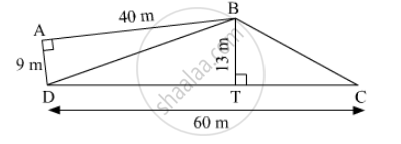SSC (English Medium) Class 8Maharashtra State Board
Share

# Some Measures Are Given in the Adjacent Figure, Find the Area of ☐Abcd. - SSC (English Medium) Class 8 - Mathematics

ConceptArea of a Triangle by Heron’S Formula

#### Question

Some measures are given in the adjacent figure, find the area of ☐ABCD.#### Solution

Area of Δ BDC = 1/2 xx 13 xx 60 = 390 m²

Area of Δ BAD = 1/2 xx AB X AD = 1/2 xx 40 xx 9 = 180 m²

Area of ☐ABCD = Ar of Δ BDC + Ar of Δ BAD

= 390 + 180

= 570 m²

Is there an error in this question or solution?

#### APPEARS IN

Balbharati Solution for Balbharati Class 8 Mathematics (2019 to Current)
Chapter 15: Area
Practice Set 15.4 | Q: 3 | Page no. 101
Solution Some Measures Are Given in the Adjacent Figure, Find the Area of ☐Abcd. Concept: Area of a Triangle by Heron’S Formula.
S